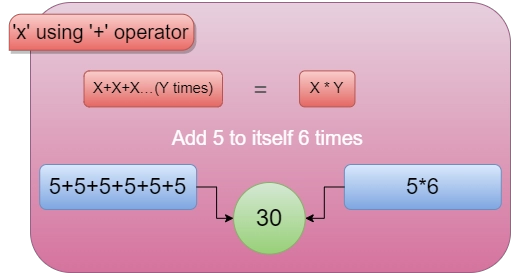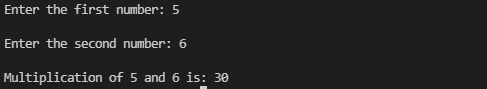New update is available. Click here to update.

# C program to multiply two numbers using plus operator

## Introduction

In this article, we will write a C program to multiply two numbers using the plus operator. To reach this goal, we need to have information about the loops in C.A loop statement allows us to execute a statement or group of statements multiple times.

Given two numbers as input from the user, we have to multiply them without using arithmetic operators like +. For this program, we are going to perform the multiplication of two numbers by repetitive addition. In other words, X * Y is the same as X + X + X … (Y times).

## Using the ‘for’ loop

A 'for' loop can be understood as a control structure that is in repetition, which allows us to efficiently write a loop which needs to be executed a specific number of times.

So, let us see how we can multiply two numbers using the plus operator using the ‘for’ loop.

Let’s see the algorithm first.

### Problem Statement

The problem statement is that we are given two integers, X and Y. We have to multiply X and Y using the plus operator.

Sample Input

Sample Output:

Explanation:### Algorithm

1. Take two numbers (X, Y) as inputs from the user.
2. Take an integer type variable for storing the result.
3. Initialize result with 0.
4. Calculate the result using the ‘for’ loop by adding the number to the result.
5. Display the result.

Implementation

``````#include <stdio.h>
int main()
{
int x, y;
int result= 0, i;

printf("Enter the first number: ");
scanf("%d",&x);

printf("\nEnter the second number: ");
scanf("%d",&y);

for(i=1;i<=y;i++) {
result += x;
}

printf("\nMultiplication of %d and %d is: %d\n" , x, y, result);
return 0;
}``````

Output:you can practice by yourself with the help of an online editor.

### Complexity Analysis

Time Complexity: O(X) or O(Y), where X and Y are the first and second number respectively.

Space Complexity: Constant space.

Also Read, Binary to Hex Converter

### What are the operators?

In mathematics and generally sometimes in computer programming, an operator is a character that represents an action; for example, x is an arithmetic operator that represents multiplication. In computer programs, one of the most familiar sets of operators, the Boolean operators, is used to work with true/false values.

### How many operators does C have?

There are five main arithmetic operators in 'C.' They are '+' for additions, '-' for subtraction, '*' for multiplication, '/' for division, and '%' for the remainder after integer division. This '%' operator is also known as the modulus operator.

### What is the difference between ++i and i++ in C?

'i++' means that when the code is executing, it will first read it and do the increment (i = i + 1) after it has been read. ++i means that when the code is executing, it will first do i=i+1 and then read it.

### What does -> mean in C?

Operation: The -> operator in C or C++ gives the value held by variable_name to structure or union variable pointer_name. Difference between Dot(.) and Arrow(->) operator: The Dot(.) operator is used to normally access members of a structure or union.

### What is %d in C programming?

In C programming language, %i and %d are known as format specifiers where %i specifies the type of variable as integer and %d specifies the type variable as a decimal. In general terms, there is almost no difference in printf() function output while printing a number using %d or %i, but using scanf, the difference occurs.

## Conclusion

In this article, we have studied how to multiply two numbers using the plus operator. We discussed two ways to do that. We hope that this article has provided you with the help to enhance your knowledge regarding programming in C language and if you would like to learn more, check out our articles on jump statements in C/C++bitwise operators in C/C++ and C vs C++.

Refer to our guided paths on Codestudio to learn more about DSA, Competitive Programming, JavaScript, System Design, etc. Enrol in our courses and refer to the mock test and problems available, Take a look at the interview experiences and interview bundle for placement preparations.

Do upvote our blog to help other ninjas grow.

Merry Learning!